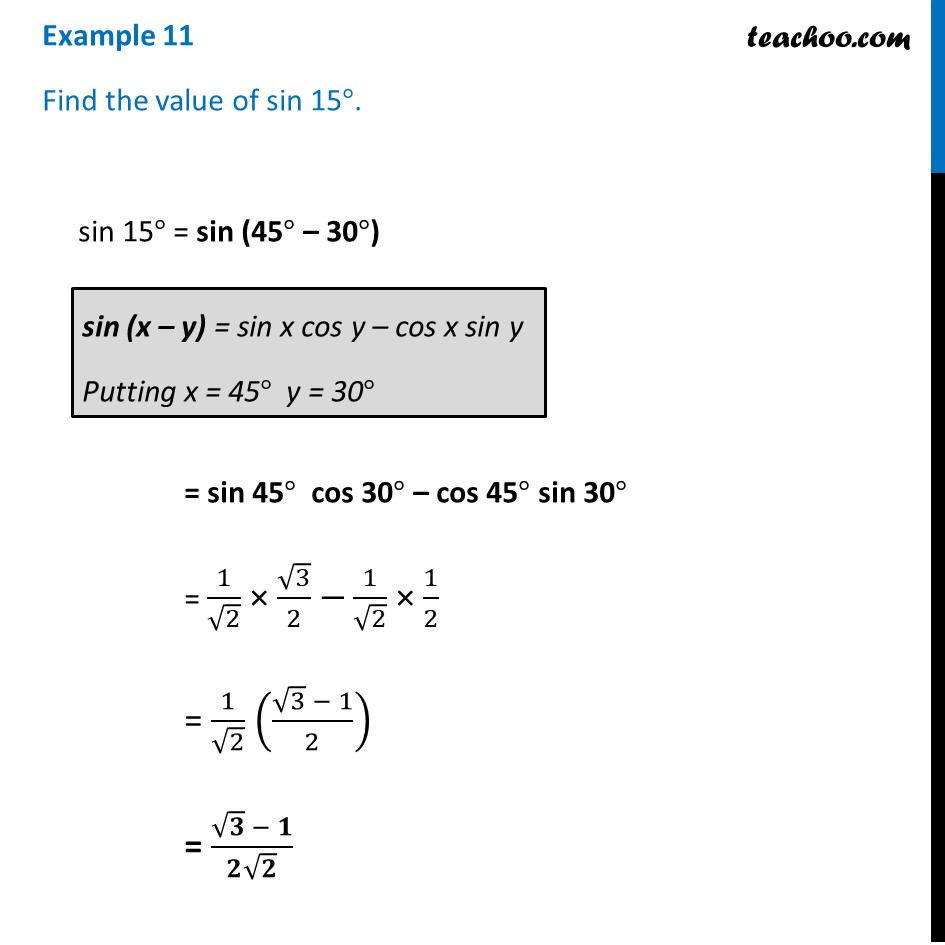Examples

Chapter 3 Class 11 Trigonometric Functions
Serial order wiseLearn in your speed, with individual attention - Teachoo Maths 1-on-1 Class

### Transcript

Example 11 Find the value of sin 15°. sin 15° = sin (45° – 30°) = sin 45° cos 30° – cos 45° sin 30° = 1/√2 × √3/2 −1/√2 × 1/2 = 1/√2 ((√3 − 1)/2) = (√𝟑 − 𝟏)/(𝟐√𝟐)